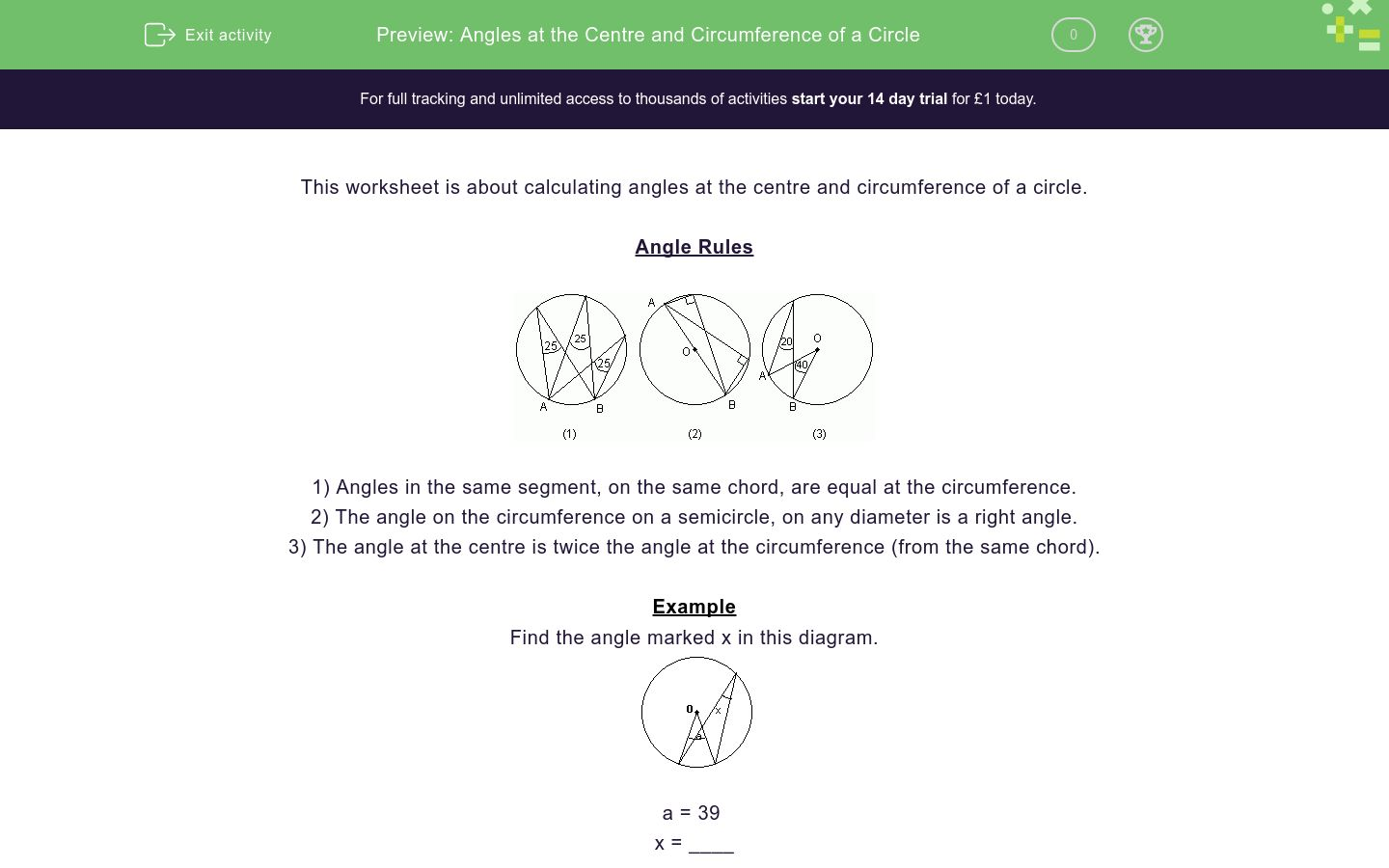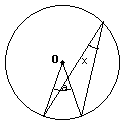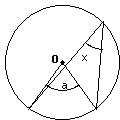# Angles at the Centre and Circumference of a Circle

In this worksheet, students resolve problems with angles at the centre and circumference of a circle.Key stage:  KS 4

Curriculum topic:  Geometry and Measures

Curriculum subtopic:  Identify and Apply Circle Definitions and Properties

Difficulty level:### QUESTION 1 of 10

This worksheet is about calculating angles at the centre and circumference of a circle.

Angle Rules1) Angles in the same segment, on the same chord, are equal at the circumference.

2) The angle on the circumference on a semicircle, on any diameter is a right angle.

3) The angle at the centre is twice the angle at the circumference (from the same chord).

Example

Find the angle marked x in this diagram.a = 39
x = ____

x is half of a, according to rule 3 above.

x = 39 ÷ 2 = 19.5 degrees

Find the angle marked x in this diagram.  All angles shown are in degrees.

(Just write the number)a = 72
x = ____

Find the angle marked x in this diagram.  All angles shown are in degrees.

(Just write the number)a = 35
x = ____

Find the angle marked x in this diagram.  All angles shown are in degrees.

(Just write the number)a = 14
x = ____

Find the angle marked x in this diagram.  All angles shown are in degrees.

(Just write the number)a = 24
x = ____

Find the angle marked x in this diagram.  All angles shown are in degrees.

(Just write the number)a = 111
x = ____

Find the angle marked x in this diagram.  All angles shown are in degrees.

(Just write the number)a = 116
x = ____

Find the angle marked x in this diagram.  All angles shown are in degrees.

(Just write the number)a = 29
x = ____

Find the angle marked x in this diagram.  All angles shown are in degrees.

(Just write the number)a = 116
x = ____

Find the angle marked x in this diagram.  All angles shown are in degrees.

(Just write the number)a = 41
x = ____

Find the angle marked x in this diagram.  All angles shown are in degrees.

(Just write the number)a = 39
x = ____

• Question 1

Find the angle marked x in this diagram.  All angles shown are in degrees.

(Just write the number)a = 72
x = ____

36
EDDIE SAYS
x is half of a
• Question 2

Find the angle marked x in this diagram.  All angles shown are in degrees.

(Just write the number)a = 35
x = ____

35
EDDIE SAYS
x and a are equal angles
• Question 3

Find the angle marked x in this diagram.  All angles shown are in degrees.

(Just write the number)a = 14
x = ____

14
EDDIE SAYS
x and a are equal angles
• Question 4

Find the angle marked x in this diagram.  All angles shown are in degrees.

(Just write the number)a = 24
x = ____

24
EDDIE SAYS
x and a are equal angles
• Question 5

Find the angle marked x in this diagram.  All angles shown are in degrees.

(Just write the number)a = 111
x = ____

55.5
EDDIE SAYS
x is half of a
• Question 6

Find the angle marked x in this diagram.  All angles shown are in degrees.

(Just write the number)a = 116
x = ____

58
EDDIE SAYS
x is half of a
• Question 7

Find the angle marked x in this diagram.  All angles shown are in degrees.

(Just write the number)a = 29
x = ____

29
EDDIE SAYS
x and a are equal angles
• Question 8

Find the angle marked x in this diagram.  All angles shown are in degrees.

(Just write the number)a = 116
x = ____

58
EDDIE SAYS
x is half of a
• Question 9

Find the angle marked x in this diagram.  All angles shown are in degrees.

(Just write the number)a = 41
x = ____

20.5
EDDIE SAYS
x is half of a
• Question 10

Find the angle marked x in this diagram.  All angles shown are in degrees.

(Just write the number)a = 39
x = ____